# Axonometry

### The method of parallel projection

 - Let a plane Π and a line a, which are not parallel, be given in space. The mapping that corresponds to every point T in space a point T, which is the intersection of the plane Π with a line incident with T and parallel to the line a, is called parallel projection to a plane Π in direction a. - Lines parallel to line a are called rays of projection and the plane Π is called the projection plane. If the rays of projection are not perpendicular to the projection plane, then the parallel projection is called oblique parallel projection.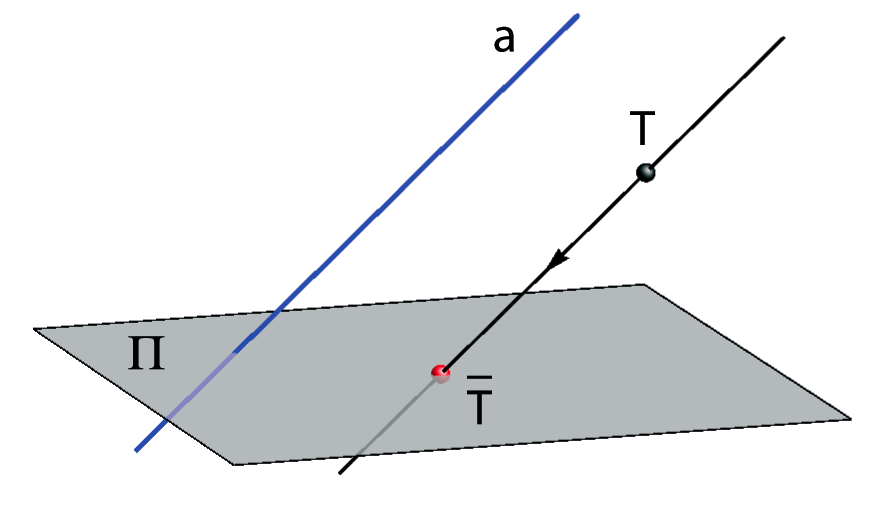#### Some properties of the oblique parallel projection

• A projection of a line, which is not a ray of projection,is a line.
• Parallel lines, which are not rays of projection, are an invariance of oblique parallel projection.

• The length of a projection A B of a line segment (not parallel to the ray of projection) can be longer, equal or shorter than the length of the line segment AB.
• A line segment is projected in a line segment of equal length if the line segment is parallel to the projection plane.
• Segment ratio (if a line segment does not lie on a ray of projection) is an invariance of parallel projection.

• The angle between two lines is equal to the angle between its projections if the lines are parallel to the projection plane.
•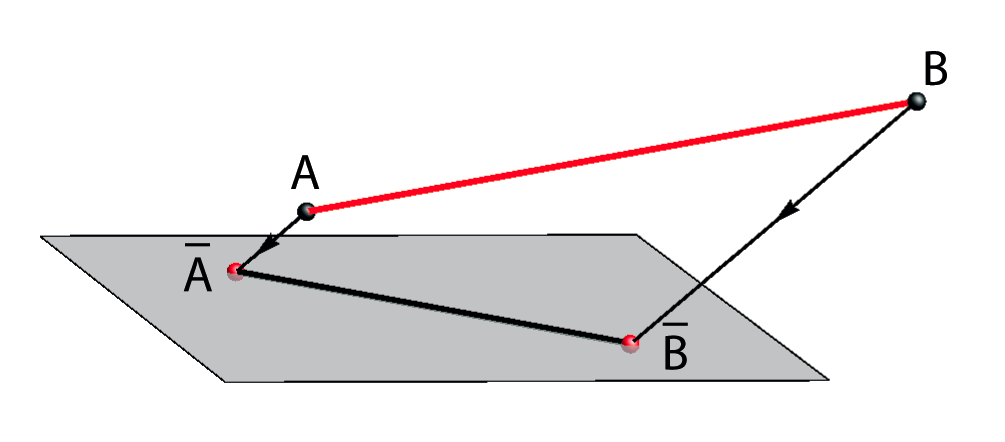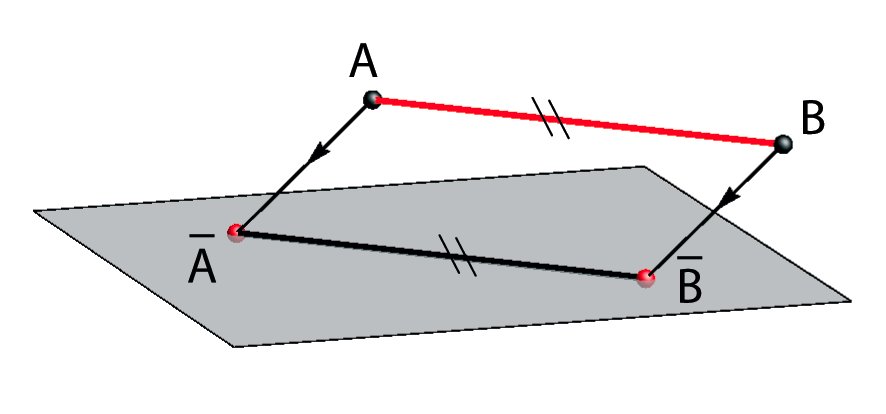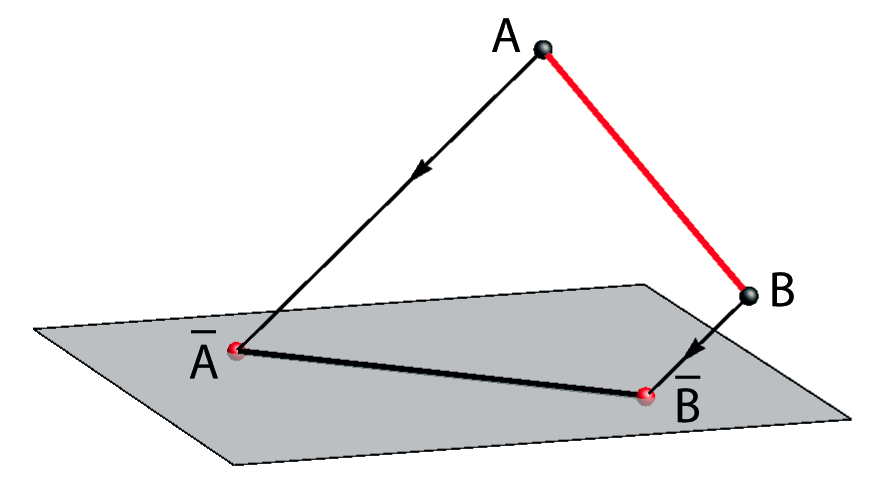d(A,B) < d(A,B) d(A,B) = d(A,B) d(A,B) > d(A,B)

### Plane and spatial three-frame

 Three line segments OA, OB and OC in space that are of unit length and are perpendicular to each other are called orthonormal three-frame. (Play the animation on the right). Let O, A, B and C be four different non-collinear points in a plane. Plane figure determined by three line segments O A, O B and O C is called the plane three-frame and is denoted by O(A,B,C). (See the lower figure.)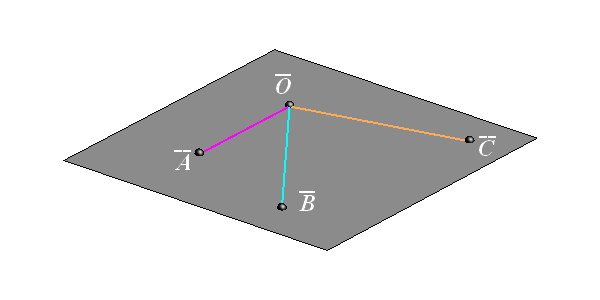for starting the animation: right click -> PLAY

 Let O(A,B,C) be the orthonormal three-frame in space for which no line segment is parallel to the ray of projection. Then the following is valid: Oblique parallel projection of the three-frame O(A,B,C) is the plane three-frame O(A,B,C) in the projection plane. A plane three-frame O(A,B,C) obtained by this way is also called Pohlke's three-frame. for starting the animation: right click -> PLAY

### Pohlke's theorem (Fundamental theorem of axonometry)

 There exists an oblique parallel projection for which every plane three-frame O(A,B,C) can be a projection of a spatial orthonormal three-frame O(A,B,C). The proof of this theorem is not simple and it will not be shown here.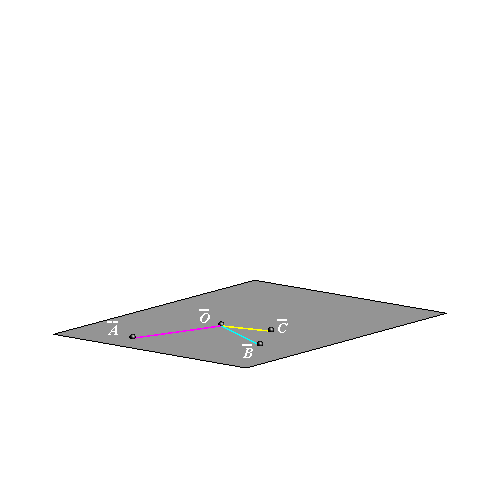## Axonometry (Rectangle Projection)

Let O(x,y,z) be the Cartesian coordinate system and let points X, Y, Z be incident with the coordinate axes (X ∈ x, Y ∈ y, Z ∈ z) where the following is valid
d(O,X) = d(O,Y) = d(O,Z) = 1, i.e. O(X,Y,Z) is an orthonormal three-frame.

If a spatial object connected with a suitably chosen coordinate system O(x,y,z) is projected (together with the coordinate axes and unit line segment on it)
with the parallel projection onto a plane, then an axonometric image of the object is obtained.

The method of this projection is called axonometry.
If the direction of the projection is not orthogonal to the image plane (projection plane) then the method is called oblique axonometry.

According to Pohlke's theorem we can choose any plane three-frame in the image plane O(X,Y,Z), i.e. four non-collinear points, and consider it to be a parallel projection of the orthonormal three-frame O(X,Y,Z).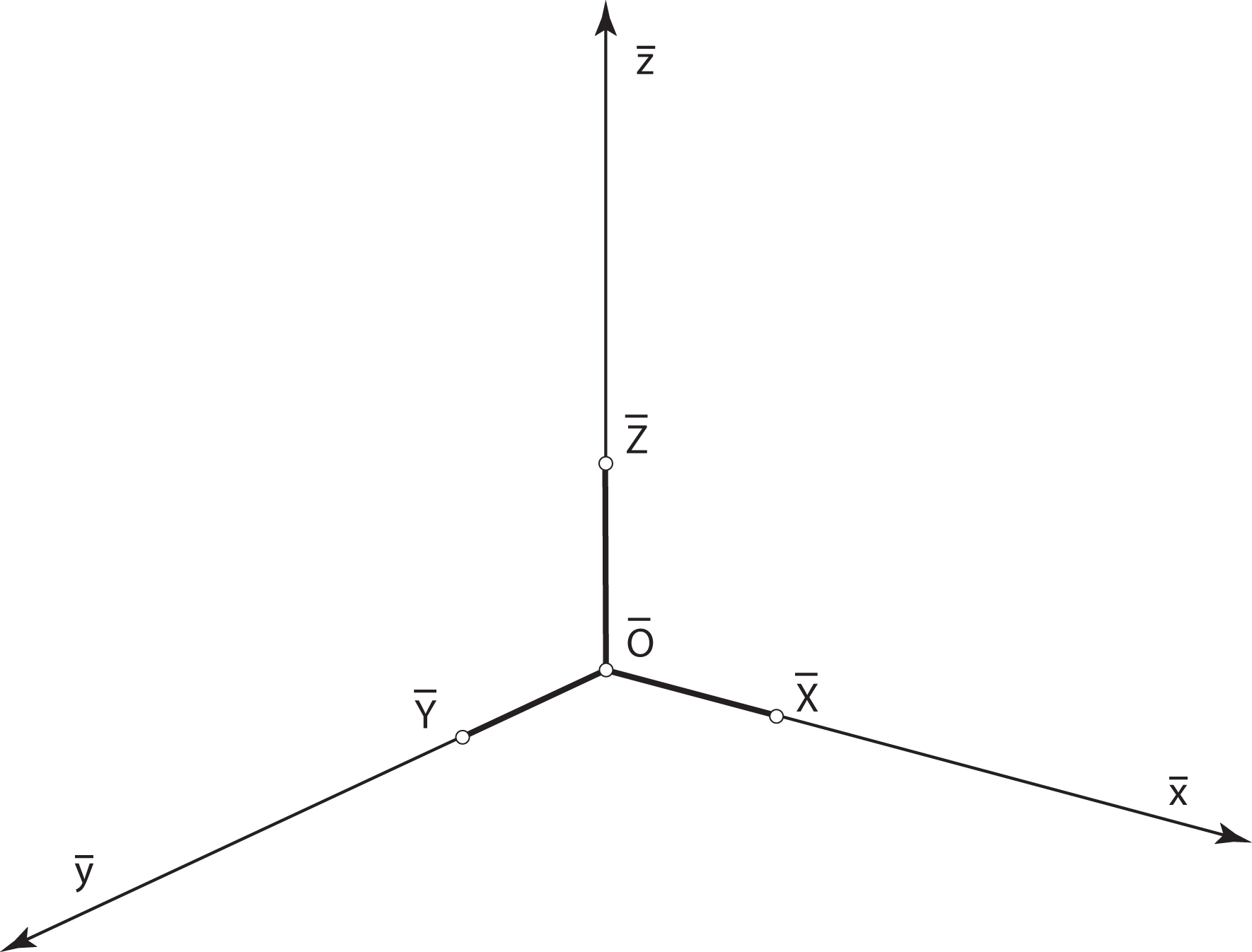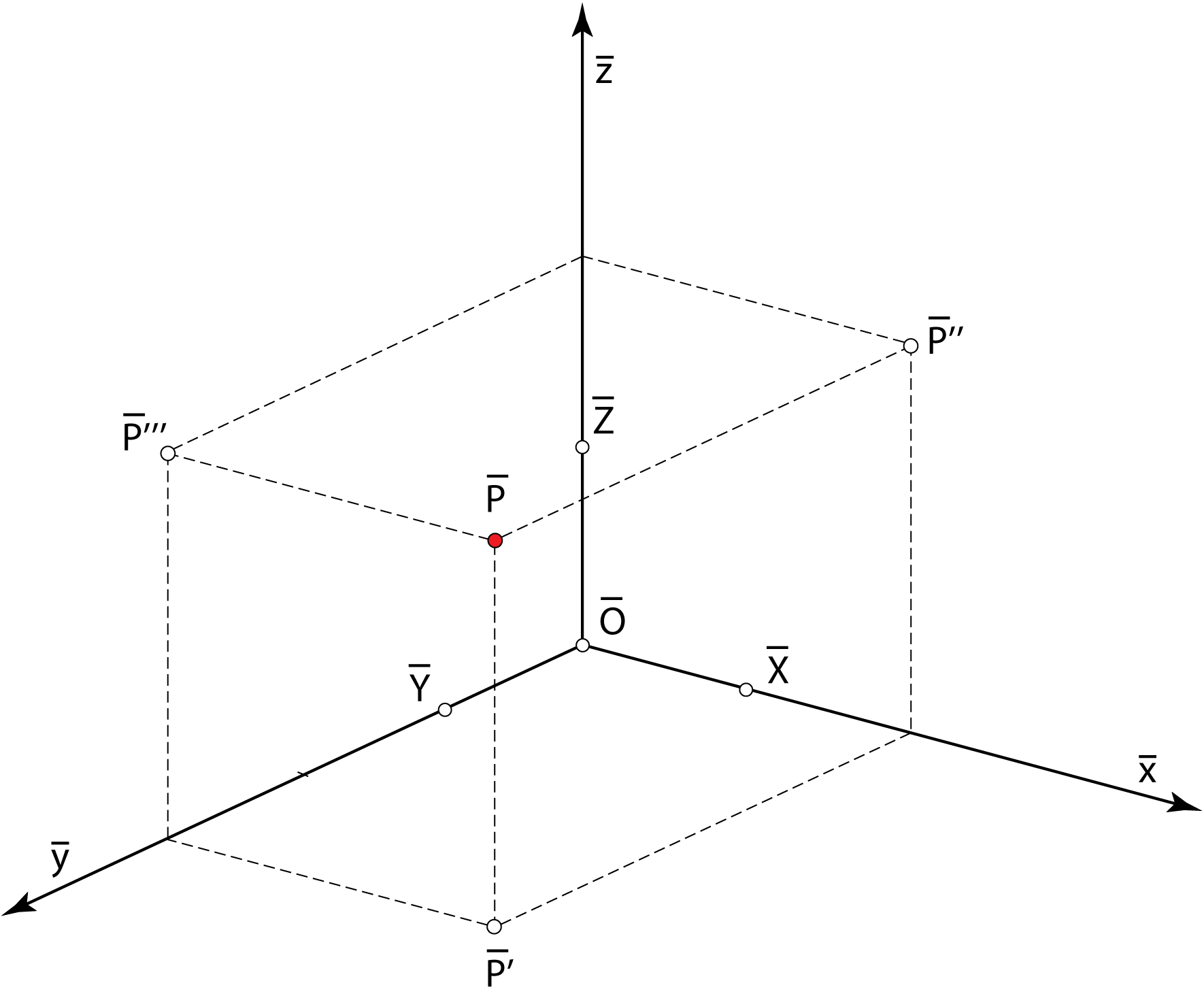Axonometric image of a three-frame.The y-axis is usually put in the vertical position. Axonometric image of the point P(2,3,2).

Pohlke's theorem provides that the projection of the coordinate axes and the unit lengths on it can be arbitrary chosen (so that it is suitable for the given object). According to the theorem, this can be done because there exists a parallel projection that projects the spatial orthonormal three-frame exactly into the chosen position in the image plane.
If d(O,X) = d(O,Y) = d(O,Z) = 1, then the axonometry is called isometry.

Exercise:
Construct the oblique axonometry of the objects given with its top view and front view.
Also construct them with isometry.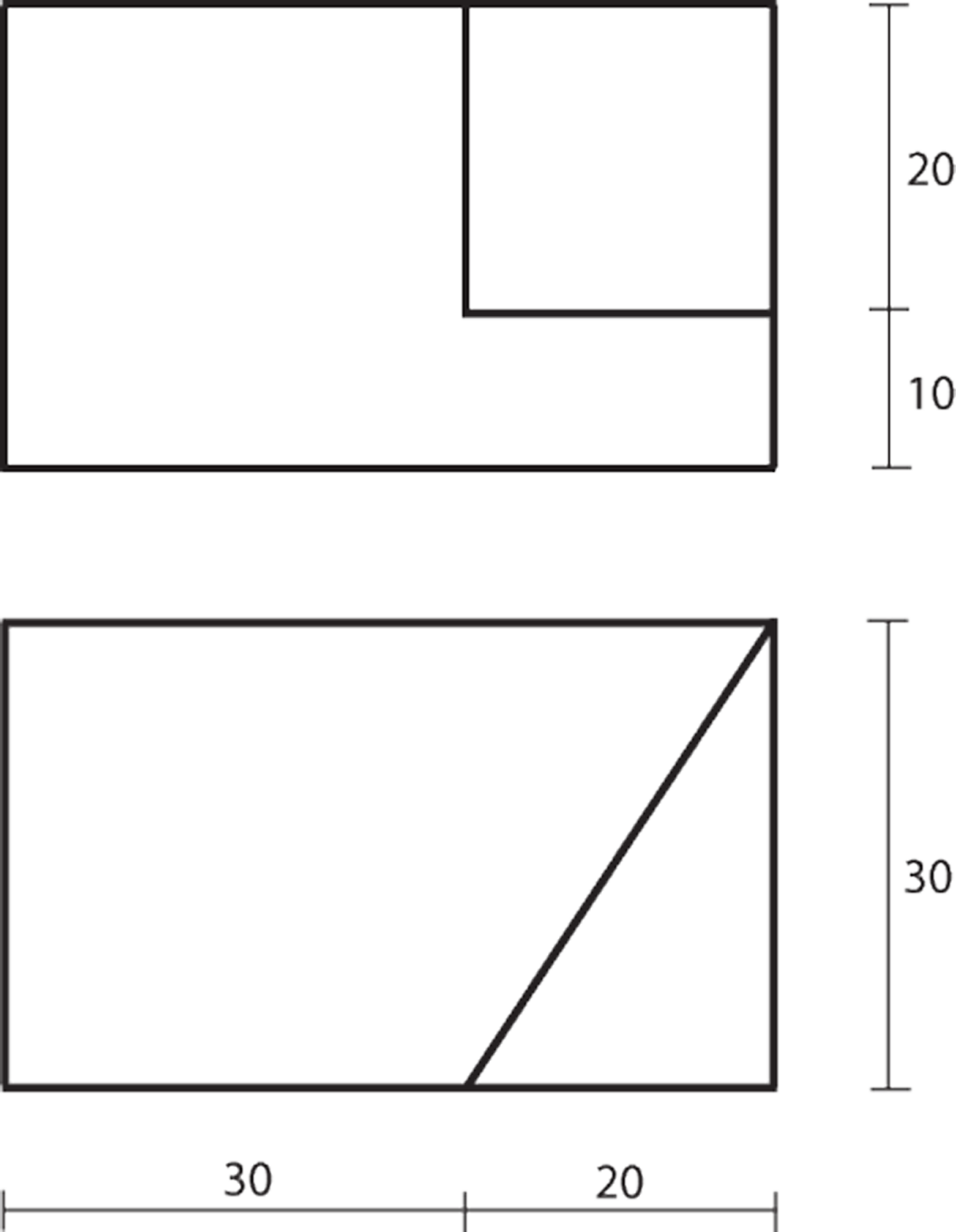Created by Sonja Gorjanc - 3DGeomTeh - Developing project of the University of Zagreb
Translated by Helena Halas and Iva Kodrnja